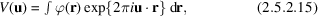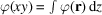International
Tables for
Crystallography
Volume B
Reciprocal space
Edited by U. Shmueli

International Tables for Crystallography (2010). Vol. B, ch. 2.5, pp. 301-302   | 1 | 2 |

## Section 2.5.2.4. Scattering of electrons by crystals; approximations

J. M. Cowleya

#### 2.5.2.4. Scattering of electrons by crystals; approximations

| top | pdf |

The forward-scattering approximation to the many-beam dynamical diffraction theory outlined in Chapter 5.2provides the basis for the calculation of diffraction intensities and electron-microscope image contrast for thin crystals. [See Cowley (1995), Chapter 5.2and IT C (2004) Sections 4.3.6and 4.3.8.] On the other hand, there are various approximations which provide relatively simple analytical expressions, are useful for the determination of diffraction geometry, and allow estimates to be made of the relative intensities in diffraction patterns and electron micrographs in favourable cases.

 (a) The kinematical approximation, derived in Section 2.5.2.2from the first Born approximation, is analagous to the corresponding approximation of X-ray diffraction. It assumes that the scattering amplitudes are directly proportional to the three-dimensional Fourier transform of the potential distribution,.so that the potential distributiontakes the place of the charge-density distribution,, relevant for X-ray scattering. The validity of the kinematical approximation as a basis for structure analysis is severely limited. For light-atom materials, such as organic compounds, it has been shown by Jap & Glaeser (1980)that the thickness for which the approximation gives reasonable accuracy for zone-axis patterns from single crystals is of the order of 100 Å for 100 keV electrons and increases, approximately as, for higher energies. The thickness limits quoted for polycrystalline samples, having crystallite dimensions smaller than the sample thickness, are usually greater (Vainshtein, 1956). For heavy-atom materials the approximation is more limited since it may fail significantly for single heavy atoms. (b) The phase-object approximation (POA), or high-voltage limit, is derived from the general many-beam dynamical diffraction expression, equation (5.2.13.1), Chapter 5.2, by assuming the Ewald sphere curvature to approach zero. Then the scattering by a thin sample can be expressed by multiplying the incoming wave amplitude by the transmission functionwhereis the projection of the potential distribution of the sample in the z direction, the direction of the incident beam. The diffraction-pattern amplitudes are then given by two-dimensional Fourier transform of (2.5.2.16). This approximation is of particular value in relation to the electron microscopy of thin crystals. The thickness for its validity for 100 keV electrons is within the range 10 to 50 Å, depending on the accuracy and spatial resolution involved, and increases with accelerating voltage approximately as. In computational work, it provides the starting point for the multislice method of dynamical diffraction calculations (IT C, 2004, Section 4.3.6.1). (c) The two-beam approximation for dynamical diffraction of electrons assumes that only two beams, the incident beam and one diffracted beam (or two Bloch waves, each with two component amplitudes), exist in the crystal. This approximation has been adapted, notably by Hirsch et al. (1965), for use in the electron microscopy of inorganic materials. It forms a convenient basis for the study of defects in crystals having small unit cells (metals, semiconductors etc.) and provides good preliminary estimates for the determination of crystal thicknesses and structure amplitudes for orientations well removed from principal axes, and for electron energies up to 200–500 keV, but it has decreasing validity, even for favourable cases, for higher energies. It has been used in the past as an extinction correction' for powder-pattern intensities (Vainshtein, 1956). (d) The Bethe second approximation, proposed by Bethe (1928)as a means for correcting the two-beam approximation for the effects of weakly excited beams, replaces the Fourier coefficients of potential by the Bethe potentials'Use of these potentials has been shown to account well for the deviations of powder-pattern intensities from the predictions of two-beam theory (Horstmann & Meyer, 1965) and to predict accurately the extinctions of Kikuchi lines at particular accelerating voltages due to relativistic effects (Watanabe et al., 1968), but they give incorrect results for the small-thickness limit.

### References

International Tables for Crystallography (2004). Vol. C. Mathematical, Physical and Chemical Tables, edited E. Prince, 3rd ed. Dordrecht: Kluwer Academic Publishers.
Bethe, H. A. (1928). Theorie der Beugung von Elektronen an Kristallen. Ann. Phys. (Leipzig), 87, 55–129.
Cowley, J. M. (1995). Diffraction Physics, 3rd ed. Amsterdam: North-Holland.
Hirsch, P. B., Howie, A., Nicholson, R. B., Pashley, D. W. & Whelan, M. J. (1965). Electron Microscopy of Thin Crystals. London: Butterworths.
Horstmann, M. & Meyer, G. (1965). Messung der Elektronenbeugungs­intensitaten polykristalliner Aluminium schichten bei tiefer Temperatur und Vergleich mit der dynamischen Theorie. Z. Phys. 182, 380–397.
Jap, B. K. & Glaeser, R. M. (1980). The scattering of high-energy electrons. II. Quantitative validity domains of the single-scattering approximations for organic crystals. Acta Cryst. A36, 57–67.
Vainshtein, B. K. (1956). Structure Analysis by Electron Diffraction. Moscow: Akad. Sci. USSR.
Watanabe, D., Uyeda, R. & Kogiso, M. (1968). An apparent variation of structure factors for electrons with accelerating voltage. An observation through Kikuchi patterns. Acta Cryst. A24, 249–250.